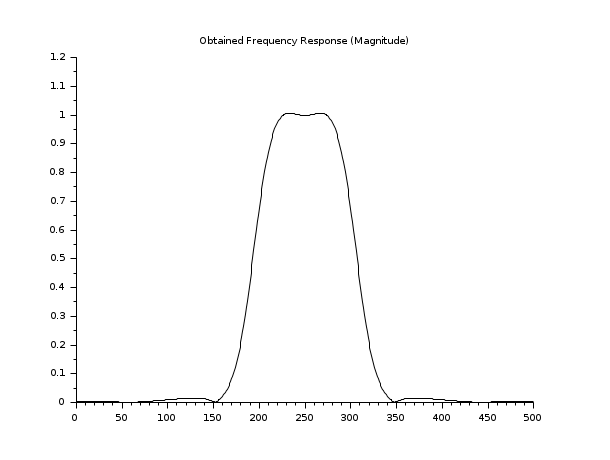Change language to:
English - 日本語 - Português - Русский

See the recommended documentation of this function

Aide de Scilab >> Traitement du Signal > filters > yulewalk

# yulewalk

least-square filter design

### Calling Sequence

`Hz = yulewalk(N,frq,mag)`

### Arguments

N

integer (order of desired filter)

frq

real row vector (non-decreasing order), frequencies.

mag

non negative real row vector (same size as frq), desired magnitudes.

Hz

filter `B(z)/A(z)`

### Description

Hz = yulewalk(N,frq,mag) finds the N-th order iir filter

```n-1         n-2
B(z)   b(1)z     + b(2)z    + .... + b(n)
H(z)= ---- = ---------------------------------
n-1       n-2
A(z)    z   + a(2)z    + .... + a(n)```

which matches the magnitude frequency response given by vectors frq and mag. Vectors frq and mag specify the frequency and magnitude of the desired frequency response. The frequencies in frq must be between 0.0 and 1.0, with 1.0 corresponding to half the sample rate. They must be in increasing order and start with 0.0 and end with 1.0.

### Examples

```f=[0,0.4,0.4,0.6,0.6,1];
H=[0,0,1,1,0,0];
Hz=yulewalk(8,f,H);
fs=1000;
fhz = f*fs/2;
clf(0);
xset('window',0);
plot2d(fhz',H');
xtitle('Desired Frequency Response (Magnitude)')
[frq,repf]=repfreq(Hz,0:0.001:0.5);
clf(1);
xset('window',1);
plot2d(fs*frq',abs(repf'));
xtitle('Obtained Frequency Response (Magnitude)')```Report an issue << window filters zpbutt >>